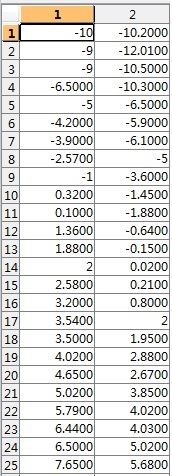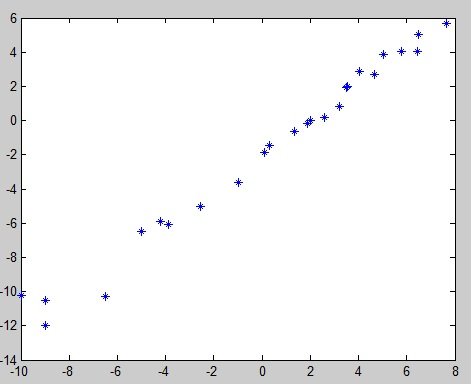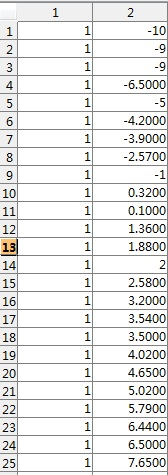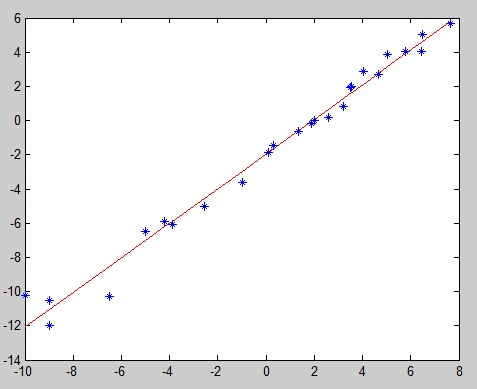###(a)                                                                                  (b)

图1  样本(a) ; 样本分布 (b)

（这里样本维度是2，故k=1)

(1)图2 扩展后的样本的向量表示(特征表示,)

(2)

1. 随机初始化 //如全为0.1

2. 重复直至收敛：

3.             以(2)式计算梯度

4.

function W = Gradient_descent(X,Y)
tX = X;%tX的作用只是为了创建detaW时使用
W = 0.1*ones(size(X,2)+1,1);
X = [ones(size(X,1),1),X];
k = 0;%迭代次数
while true
detaW = zeros(size(tX,2)+1,1);
O = X*W;
detaW = detaW + 0.00005*X'*(Y - O);%0.00005是学习速率
W = W + detaW;
k = k+1;
if 1/2*( norm(Y - X*W) )^2 < 5 || k>10000 %如果误差小于5或者迭代次数大于10000则停止
break;
end
fprintf('iterator times %d, error %f\n',k,1/2*( norm(Y - X*W) )^2);
end©️2019 CSDN 皮肤主题: 大白 设计师: CSDN官方博客